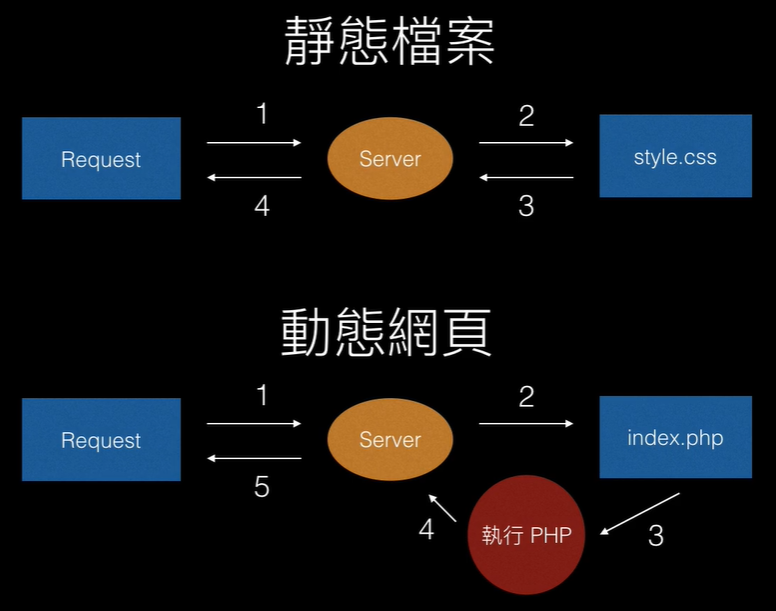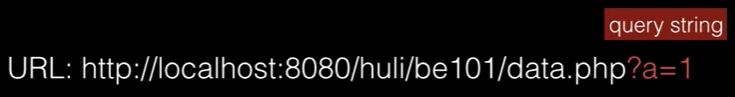# PHP 語法基礎

### 常用的三種動態網頁語言有

1. ASP (Active Server Pages) : Microsoft開發的動態網頁語言，只能執行於微軟的伺服器產品
3. JSP (Java Server Pages) : 適合基於 JAVA 技術的 JSP（結合 JavaBean 和 EJB ）

## XAMPP

XAMPP 是一個把 Apache 網頁伺服器與 PHP、Perl 及 MariaDB 集合在一起的安裝包，允許用戶可以在自己的電腦上輕易的建立網頁伺服器。使用 XAMPP ，PHP 檔案都會放在 htdocs。
htdocs 是 host documents 的縮寫。直接翻譯就是主機文件。Hosts 文件主要作用是定義 IP 地址和主機名的映射關係，是一個映射 IP 地址和主機名的規定。

• <?php?> 包起來

<?php
把 PHP 包在裡面
?>

• 變數宣告，用 $字號來表示 javaScript : var a = 1; php : $a = 1;

• 字串串接
和其他語言比較不一樣的是用 . 接起來

<?php
$a = "aaaaaaaaa";$b = "bbbbbbbbb";
echo $a .$b;
?>

• 迴圈 : 變數要 $開頭 <?php for($i=1; $i<=10;$i++) {
echo $i; . "<br>"; } ?>  • array <?php$arr = array(1, 2, 3, 4, 5);
echo "length:" . sizeof($arr) . "<br>"; echo$arr[sizeof($arr) - 1]; ?>  • 把 array 輸出要轉成 string var_dump : 函式的功能是用來印出變數的相關訊息(變數的型態跟值) print_r : 印出陣列（PHP Array）的內容 <?php$arr = array("gdgsdg", 2, 3, 4, 5);
var_dump($arr); print_r($arr);
?>


## 初探 PHP

XAMPP 有時候也會把一些資源暫存住，例如你改了一些檔案，重新整理發現沒變，可能原因是瀏覽器把暫存了之前那份檔案。## 從前端傳資料給後端：GET 與 POST

• QueryStringQueryString 會自動存到 data.php，你可以用 $_GET 這個特殊的變數，PHP 幫你準備好的變數 indext.php 檔 <?php echo "gogogogo"; ?> <form method="GET" action="data.php"> name: <input name="name" /> age: <input name="age" /> <input type="submit" /> </form>  data.php 檔 <?php if (!isset($_GET['name']) || !isset($_GET['age'])) { echo '資料有缺，請再次填寫<br>'; exit(); } echo "Hello" .$_GET['name'] . "<br>";
echo "Your age is" . $_GET['age'] . "<br>"; print_r($_GET);

?>


## PHP isset()和empty()的區別

echo "Your age is" . $_POST['age'] . "<br>"; print_r($_POST);
?>


$_GET$_POST 可以混用，但不建議

setcookiehttp://php.net/manual/en/function.setcookie.php
$_COOKIEhttp://php.net/manual/en/reserved.variables.cookies.php) setcookie 常用到的三個參數 : 舉例 : setcookie ($name, $value,$expire)
$name = "username";$value = $_POST['username'];$expire = time() + 3600 * 24 * 30;




2. 第三個引數 cookie 的過期時間設定為小於系統的當前時間，或是直接設為 0 就好
setcookie("username", "", 0);


## Huli 影片補充 :

die 跟 exit 這兩個 function 其實是一樣的